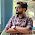## Majority Element II

Given an integer array of size n, find all elements that appear more than ⌊ n/3 ⌋ times. The algorithm should run in linear time and in O(1) space.

### Analysis:

This problem can be solved using the  Boyer-Moore majority vote algorithm.

Before we are digging into this algorithm, let's see one basic observation:
How many majority elements at most in this problem?
Explanation: At most there are 3 elements appear exactly n/3 times. So for more than n/3 times, at most there are 2 elements.

Now we discuss the Moore majority vote algorithm. Quoted from wiki:
"
The algorithm is carried out in two steps:

1. Eliminate all elements except one.

Iterating through the array of numbers, maintain a current candidate and a counter initialized to 0. With the current element x in iteration, update the counter and (possibly) the candidate:

If the counter is 0, set the current candidate to x and the counter to 1. If the counter is not 0, increment or decrement the counter based on whether x is the current candidate.

2. Determine if the remaining element is a valid majority element.

With the candidate acquired in step 1, iterate through the array of numbers and count its occurrences. Determine if the result is more than half of the sequence's length. If so, the candidate is the majority. Otherwise, the sequence doesn't contain a majority.

"

The key idea of this algorithm is "decrement" of the counter when new elements are different from all the current candidates.  This "decrement" operation is to cancel out the elements that do not appear most times in the array. Therefore, after one scan, the candidates are the elements which appear most of the times. The last  step is to check if these candidates are satisfied with the condition of more than n/3 times.

### Code (C++):

class Solution {
public:
vector<int> majorityElement(vector<int>& nums) {
vector<int> res;
int a,b;
int ca = 0;
int cb = 0;

for (int i=0;i<nums.size();i++){
if (nums[i] == a || ca == 0){
a = nums[i];
ca += 1;
}else if(nums[i] == b  || cb == 0){
b = nums[i];
cb += 1;
}else{
ca --;
cb --;
}
}

ca = 0;
cb = 0;
for (int i=0;i<nums.size();i++){
if (nums[i] == a){ ++ca; }
else if (nums[i] == b){ ++cb; }
}

if (ca > floor(nums.size()/3) ){ res.push_back(a);}
if (cb > floor(nums.size()/3)){ res.push_back(b);}

return res;
}
};


### Code (Python):

class Solution(object):
def majorityElement(self, nums):
"""
:type nums: List[int]
:rtype: List[int]
"""
res = []
a = 0
b = 0
ca = 0
cb = 0

for num in nums:
if num == a or ca == 0:
a = num
ca += 1
elif num == b or cb == 0:
b = num
cb += 1
else:
ca -= 1
cb -= 1

ca = 0
cb = 0
for num in nums:
if num == a:
ca += 1
elif num == b:
cb += 1
if ca > math.floor(len(nums)/3):
res.append(a)
if cb > math.floor(len(nums)/3):
res.append(b)

return res


1.Your code is not working for [1,2,2,3,2,1,1,3]
It needs a little modification to handle the situation when both 'a' and 'b' holds the same value.

for (int i=0;i<nums.size();i++){
if (nums[i] == a || ca == 0)
{
if(cb != 0)
{
if(nums[i] != b)
{
a = nums[i];
ca += 1;
}
}
else
{
a = nums[i];
ca += 1;
}

}

1.The Python code is actually working for your case of [1,2,2,3,2,1,1,3] n=8.
1, ca=1 a=1 cb=0 b=0
2, ca=1 a=1 cb=1 b=2
2, ca=1 a=1 cb=2 b=2
3, ca=0 a=1 cb=1 b=2
2, ca=0 a=1 cb=2 b=2
1, ca=1 a=1 cb=2 b=2
1, ca=2 a=1 cb=2 b=2
3 ca=1 a=1 cb=1 b=2

The point is that there are two conditions in the Yu's code. It rules out the possibility that 'a' and 'b' holds the same value. It is just because of the two conditions. The code can find out majority of items which occurs [n/3].

2.The Python code is actually working for your case of [1,2,2,3,2,1,1,3] n=8.
1, ca=1 a=1 cb=0 b=0
2, ca=1 a=1 cb=1 b=2
2, ca=1 a=1 cb=2 b=2
3, ca=0 a=1 cb=1 b=2
2, ca=0 a=1 cb=2 b=2
1, ca=1 a=1 cb=2 b=2
1, ca=2 a=1 cb=2 b=2
3 ca=1 a=1 cb=1 b=2

The point is that there are two conditions in the Yu's code. It rules out the possibility that 'a' and 'b' holds the same value. It is just because of the two conditions. The code can find out majority of items which occurs [n/3].

3.if((nums[i]==a || count_a==0) && nums[i]!=b)
{
a=nums[i];
count_a+=1;
}
It works fine now.

2.Isn't the C++ code broken since a and b have not yet been initialized at the time of the first if statement comparison?

3.Correct Python code:

--snip ---
for num in nums:
if (num == a or ca == 0) and (b != num):
a = num
ca += 1
elif num == b or cb == 0:
b = num
cb += 1
else:
ca -= 1
cb -= 1
--snip--